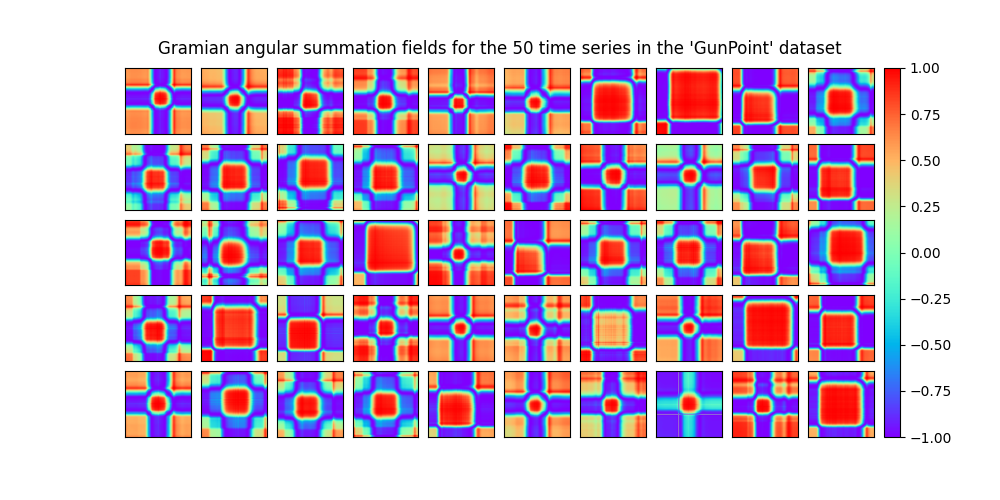# Data set of Gramian angular fields¶

A Gramian angular field is an image obtained from a time series, representing some kind of temporal correlation between each pair of values from the time series. Two methods are available: Gramian angular summation field and Gramian angular difference field. It is implemented as `pyts.image.GramianAngularField`.

In this example, we consider the training samples of the GunPoint dataset, consisting of 50 univariate time series of length 150. The Gramian angular summation field of each time series is independently computed and the 50 Gramian angular summation fields are plotted.```# Author: Johann Faouzi <johann.faouzi@gmail.com>

import matplotlib.pyplot as plt
from mpl_toolkits.axes_grid1 import ImageGrid
from pyts.image import GramianAngularField

X, _, _, _ = load_gunpoint(return_X_y=True)

# Get the Gramian angular summation fields for all the time series
gaf = GramianAngularField()
X_gaf = gaf.fit_transform(X)

# Plot the 50 Gramian angular fields
fig = plt.figure(figsize=(10, 5))

grid = ImageGrid(fig, 111, nrows_ncols=(5, 10), axes_pad=0.1, share_all=True,
cbar_mode='single')
for i, ax in enumerate(grid):
im = ax.imshow(X_gaf[i], cmap='rainbow', origin='lower', vmin=-1., vmax=1.)
grid.get_yaxis().set_ticks([])
grid.get_xaxis().set_ticks([])
plt.colorbar(im, cax=grid.cbar_axes)
ax.cax.toggle_label(True)

fig.suptitle("Gramian angular summation fields for the 50 time series in the "
"'GunPoint' dataset", y=0.92)

plt.show()
```

Total running time of the script: ( 0 minutes 4.583 seconds)

Gallery generated by Sphinx-Gallery